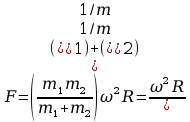# Soal Fisika Mekanika Kjlj The Velocity V Of Waves On A

1. The velocity v of waves on a vibrating string depends on tension T in the string and the mass per unit length of the string.Derive an expression for v by using the dimensional analysis method.

2. A mass m is given an initial velocity v0 up an inclined plane of angle (is greater than the angle of friction). Find the distance the mass moves up the incline, the time it take to reach this point, and the time it takes to return to its original position.

3. Consider a system of two masses and a pulley as shown in Fig. P1.10. Let m1 = 12 kg, m2 = 8 kg the mass of the pulley m = 10k, and its radius r = 10 cm.

1. Show all the forces acting on the system.

2. Calculate T1, T2, and acceleration a. Assume the pulley to be a solid dis (Idisk = mr2/2).

1. Two particles of mass m1 and m2 at a distance R from each other are under the influence of an attractive force F. If the two masses undergo uniform circular motion about each other with an angular velocity , show that1. Find the mass of the Sun, assuming that Earth moves in a circular orbit of radius 1,496×108m and completes one revolution around the Sun in one year.